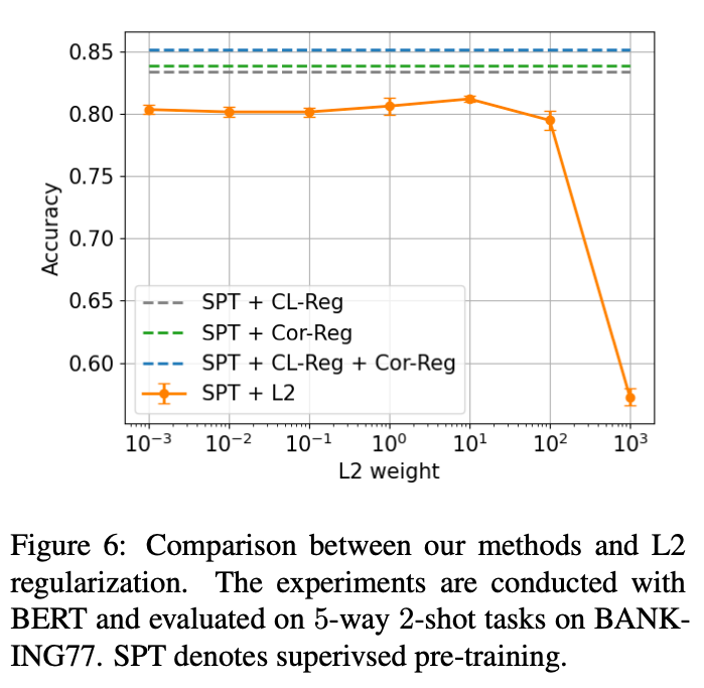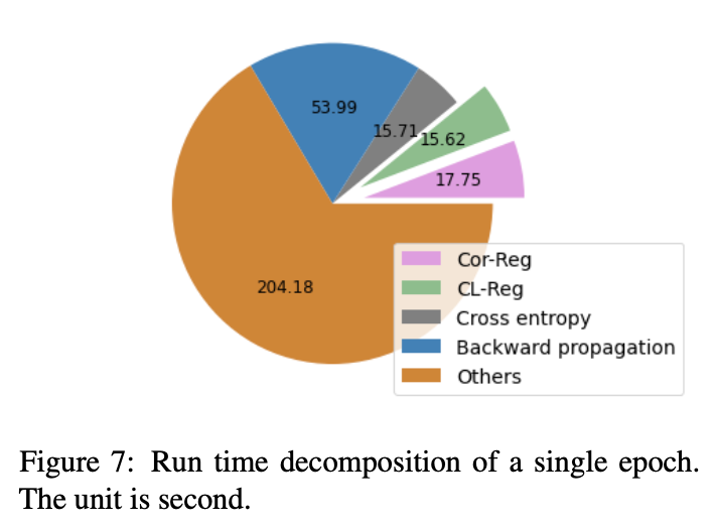# NLP 論文領讀｜改善意圖識別的語義表示：有監督預訓練中的各向同性正則化方法

## theme: smartblue

E-mail: [email protected]

## 寫在前面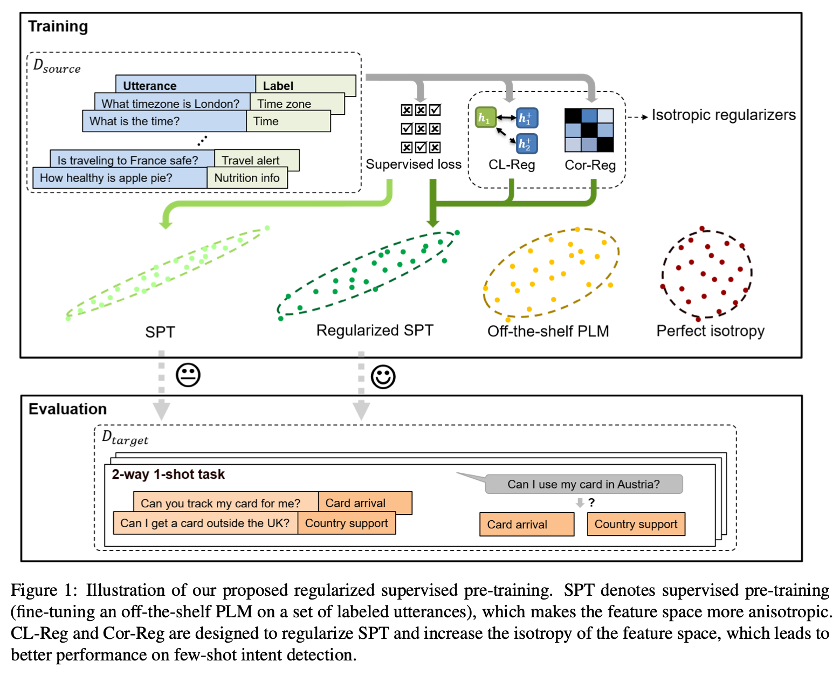\

Fine-tuning Pre-trained Language Models for Few-shot Intent Detection: Supervised Pre-training and Isotropization

Haode Zhang, Haowen Liang, Yuwei Zhang, Liming Zhan, Xiao-Ming Wu, Xiaolei Lu, Albert Y.S. Lam

Department of Computing, The Hong Kong Polytechnic University, Hong Kong S.A.R.

University of California, San Diego

Nanyang Technological University, Singapore

Fano Labs, Hong Kong S.A.R.

https://arxiv.org/abs/2205.07208

https://github.com/fanolabs/isoIntentBert-main

## Pilot Study

$\mathrm{I}(\mathbf{V})=\frac{\min {\mathbf{c} \in C} \mathbf{Z}(\mathbf{c}, \mathbf{V})}{\max {\mathbf{c} \in C} \mathbf{Z}(\mathbf{c}, \mathbf{V})}$ (1)

$\mathbf{Z}(\mathbf{c}, \mathbf{V})=\sum_{i=1}^{N} \exp \left(\mathbf{c}^{\top} \mathbf{v}_{i}\right)$ (2)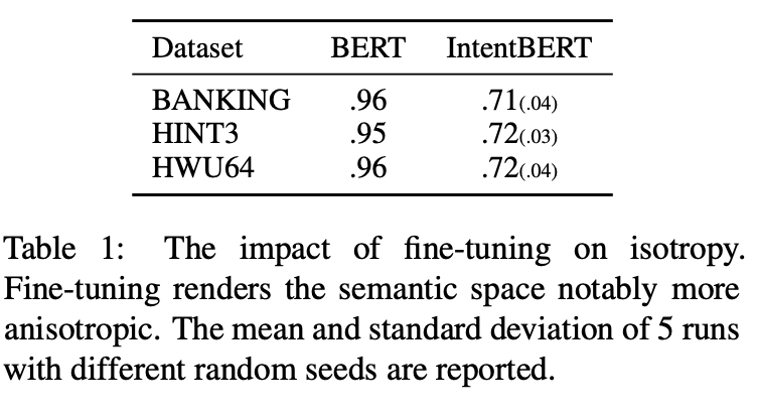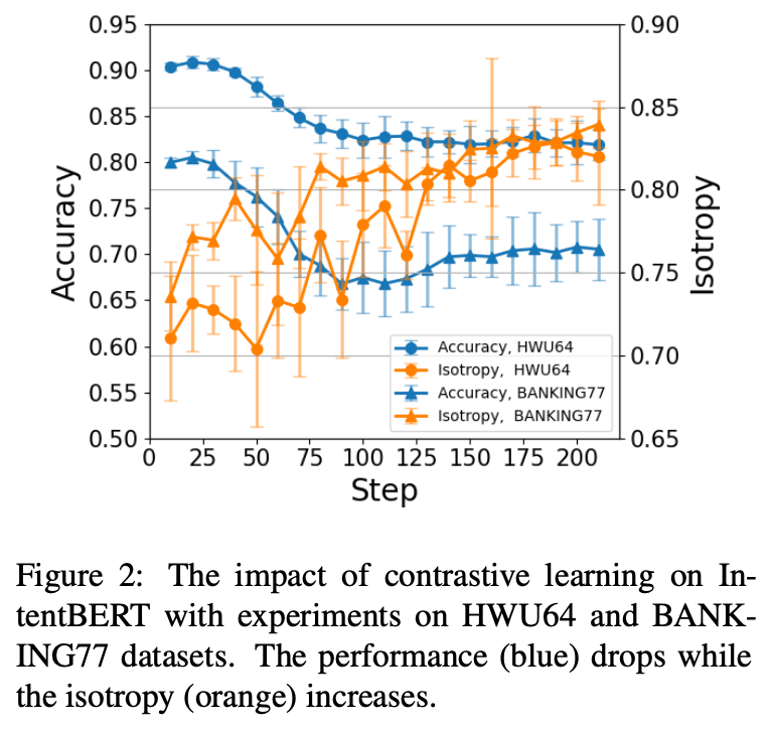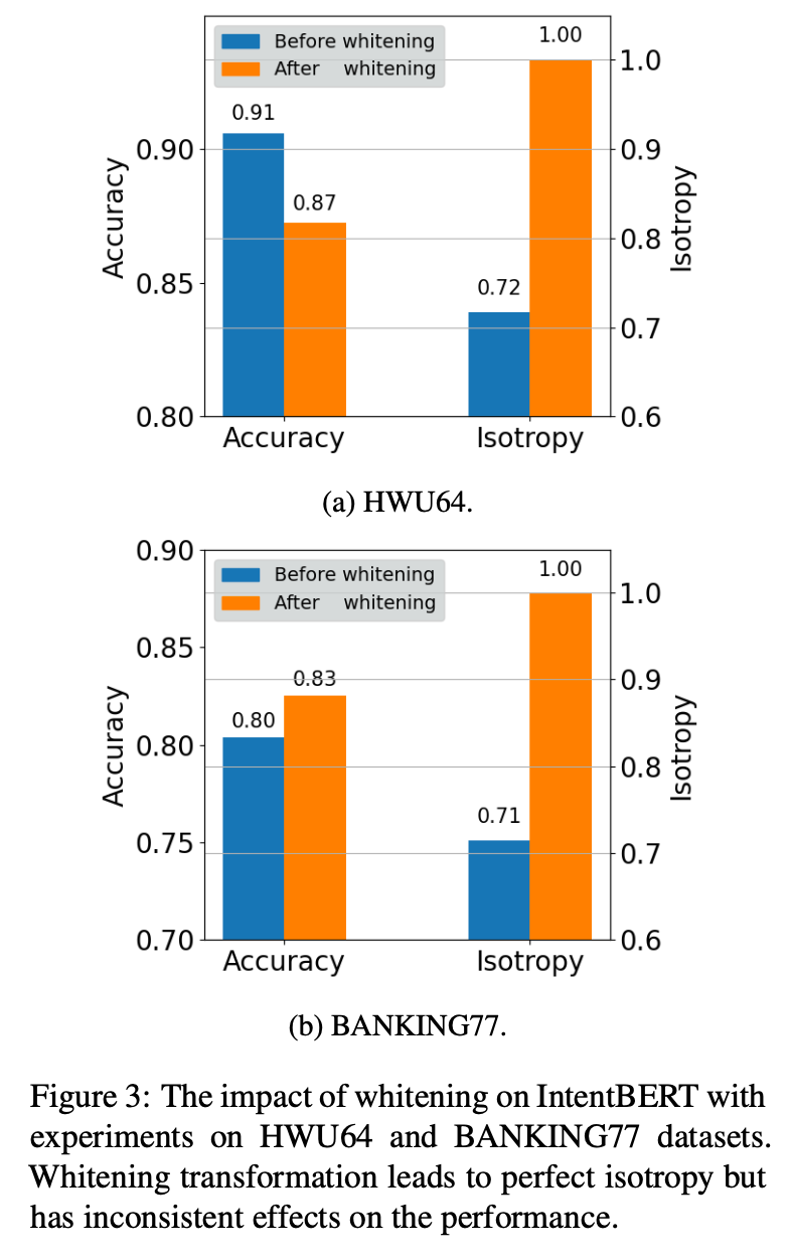• dropout-based contrastive learning 
• whitening transformation 

## Method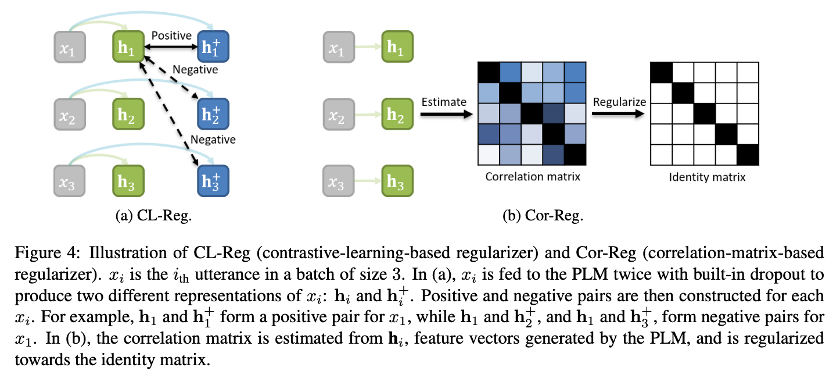### 1. Supervised Pre-training for Few-shot Intent Detection

$\mathrm{p}\left(y \mid \mathbf{h}{i}\right)=\operatorname{softmax}\left(\mathbf{W} \mathbf{h}{i}+\mathbf{b}\right) \in \mathbb{R}^{L}$ (3)

$\theta=\underset{\theta}{\arg \min } \mathcal{L}{\mathrm{ce}}\left(\mathcal{D}{\text {source }} ; \theta\right)$ (4)

### 2. Regularizing Supervised Pre-training with Isotropization

$\mathcal{L}=\mathcal{L}{\text {ce }}\left(\mathcal{D}{\text {source }} ; \theta\right)+\lambda \mathcal{L}{\text {reg }}\left(\mathcal{D}{\text {source }} ; \theta\right)$ (5)

Contrastive-learning-based Regularizer

$\mathcal{L}{\mathrm{reg}}=-\frac{1}{N{b}} \sum_{i}^{N_{b}} \log \frac{e^{\operatorname{sim}\left(\mathbf{h}{i}, \mathbf{h}{i}^{+}\right) / \tau}}{\sum_{j=1}^{N_{b}} e^{\operatorname{sim}\left(\mathbf{h}{i}, \mathbf{h}{j}^{+}\right) / \tau}}$ (6)

$x_i$ 是 $\mathcal{D}{\text {source }}$ 中的一條資料，$x_i$ 輸入 PLM 兩次，使用不同的 dropout masks 來得到兩個不同的表示 $\mathbf{h}{i}$和$\mathbf{h}{i}^{+}$，$\operatorname{sim}\left(\mathbf{h}{i}, \mathbf{h}{i}^{+}\right)$ 是餘弦相似度，因為$\mathbf{h}{i}$ 和 $\mathbf{h}{i}^{+}$ 表示同一個輸入，所以它們算是 positive pair，$\mathbf{h}{i}$ 和 $\mathbf{h}_{j}^{+}$ 就是 negative pair，前面說過，各向異性的特徵向量都擠在一起，彼此距離很近，加了正則項後，在訓練時，positive pairs 距離變近，negative pairs 距離變遠，特徵空間就更加各向同性。

Correlation-matrix-based Regularizer

$\mathcal{L}_{\mathrm{reg}}=\|\boldsymbol{\Sigma}-\mathbf{I}\|$ (7)

\begin{aligned} \mathcal{L}=\mathcal{L}{\text {ce }}\left(\mathcal{D}{\text {source }} ; \theta\right)+\lambda_{1} \mathcal{L}{\text {cl }}\left(\mathcal{D}{\text {source }} ; \theta\right)+\lambda_{2} \mathcal{L}{\text {cor }}\left(\mathcal{D}{\text {source }} ; \theta\right) \end{aligned} (8)

## 實驗設定及結果

### 1. 實驗設定

#### 資料集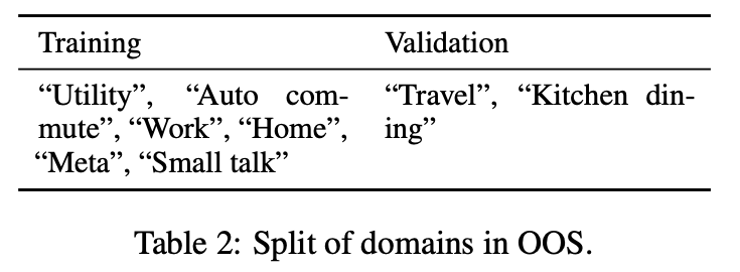• BANKING77：關於銀行服務的意圖識別資料集；
• HINT3：覆蓋 3 個領域，“Mattress Products Re- tail”、“Fitness Supplements Retail”和“Online Gaming”；
• HWU64：包含21個領域的大規模資料集。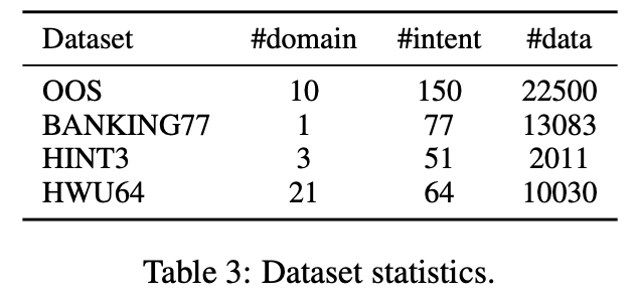#### 引數設定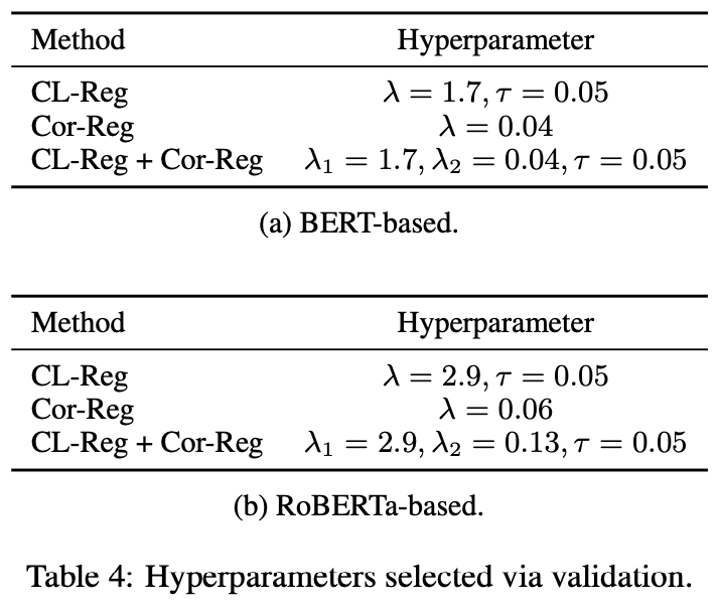#### 基線模型

• CONVBERT
• TOD-BERT
• DNNC-BERT
• USE-ConveRT
• CPFT-BERT

• RoBERTa-Freeze
• WikiHowRoBERTa
• DNNC-RoBERTa
• CPFT-RoBERTa
• IntentRoBERTa

• BERT-White
• RoBERTa-White
• IntentBERT-White
• IntentRoBERTa-White

#### 訓練細節

• Pytorch、Python
• Hugging Face的bert-base-uncased和roberta-base
• Adam 優化器，學習率 2e-05，weight decay 為 1e-03
• Nvidia RTX 3090 GPUs
• 在驗證集上 100 步沒有提升就停止訓練
• 隨機種子集合 {1, 2, 3, 4, 5}

### 2. Main Results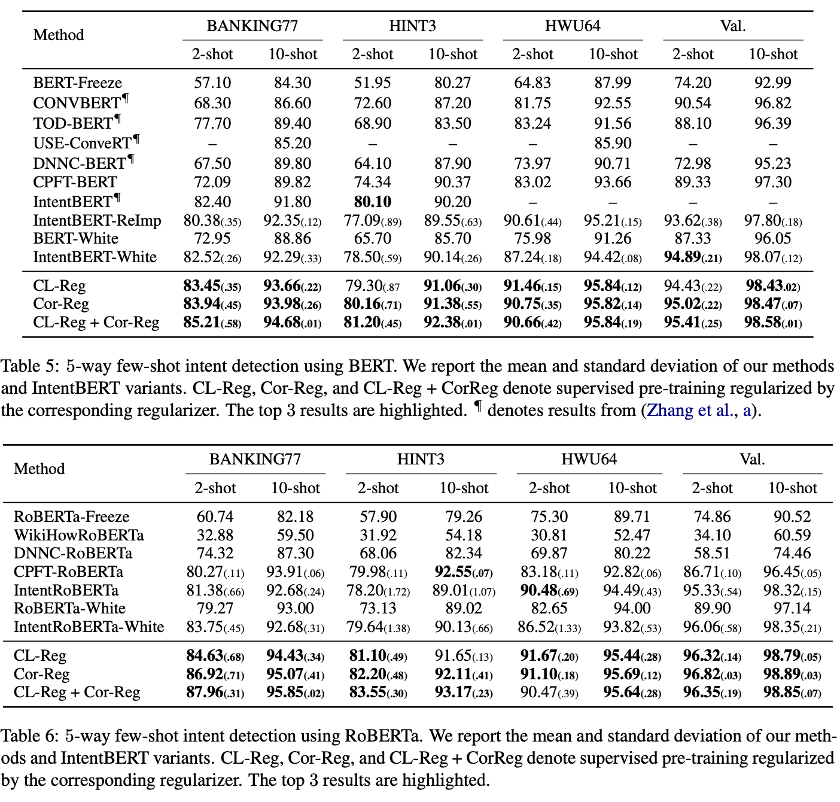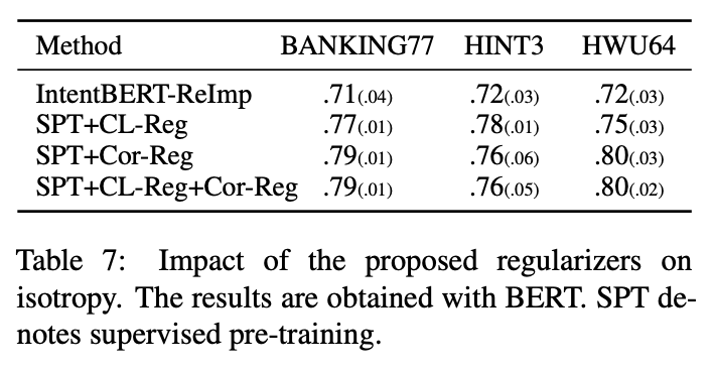### 3. 消融實驗和分析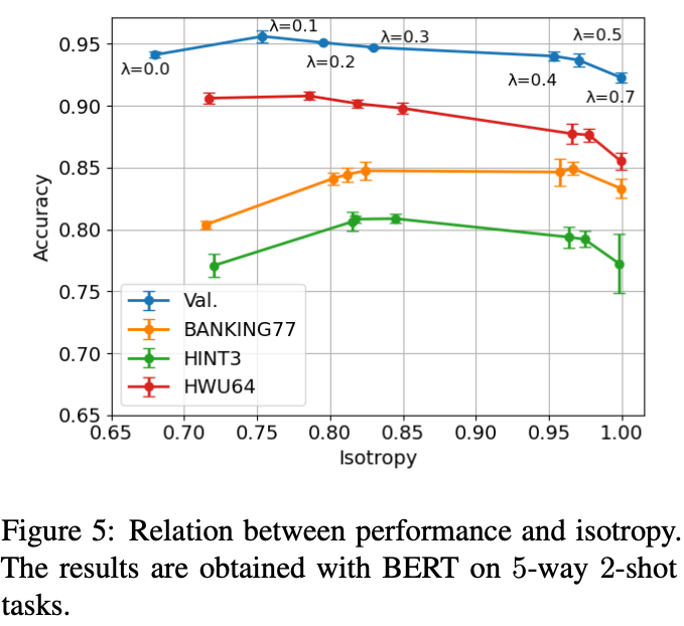Correlation matrix is better than covariance matrix as regularizer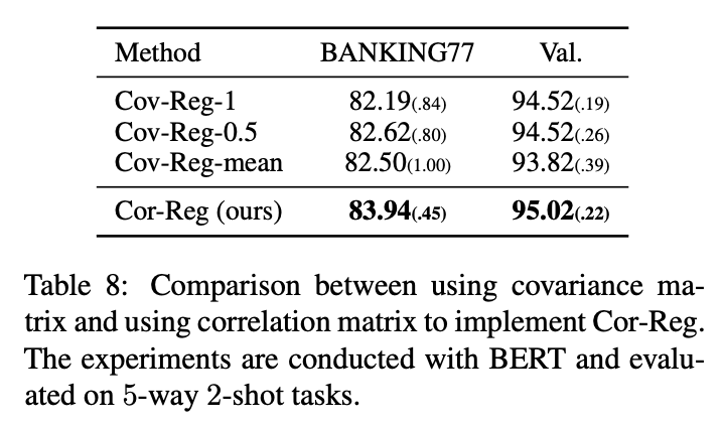The performance gain is not from the reduction in model variance

L1 和 L2 正則化可以通過減小模型方差來提升效能，而作者將表 7 裡的效能提升歸因於各向同性的改善。為了探究使用了正則項後效能的提升是來自方差還是各向同性，作者給 L2正 則項設定不同的權重，得到多個結果，但都不及 CL-Reg 和 Cor-Reg，如圖 6 所示。